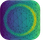#adaptive#

Adaptive: parallel active learning of mathematical functions.

adaptive is an open-source Python library designed to make adaptive parallel function evaluation simple. With adaptive you just supply a function with its bounds, and it will be evaluated at the “best” points in parameter space, rather than unnecessarily computing all points on a dense grid. With just a few lines of code you can evaluate functions on a computing cluster, live-plot the data as it returns, and fine-tune the adaptive sampling algorithm.

adaptive excels on computations where each function evaluation takes at least ≈50ms due to the overhead of picking potentially interesting points.

Run the adaptive example notebook live on Binder to see examples of how to use adaptive or visit the tutorial on Read the Docs.

Tip

Start with the 1D function learning tutorial.